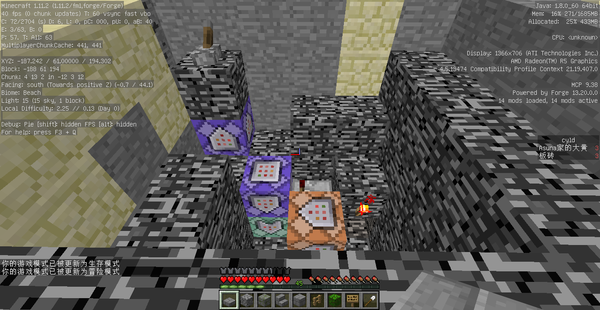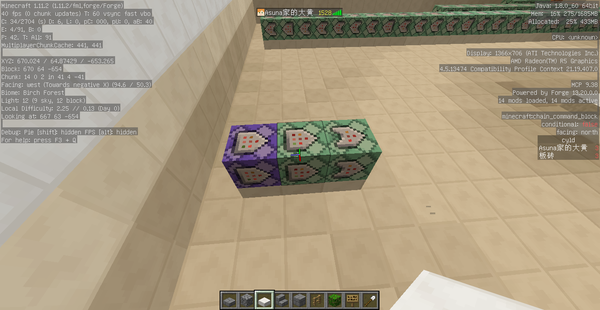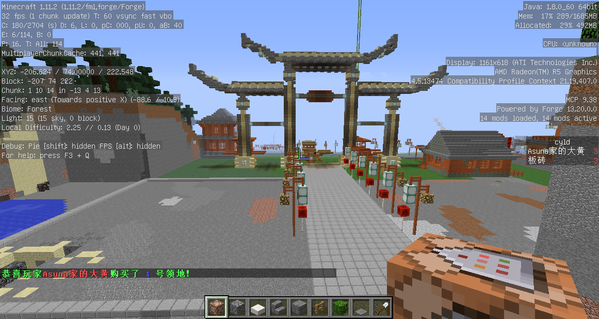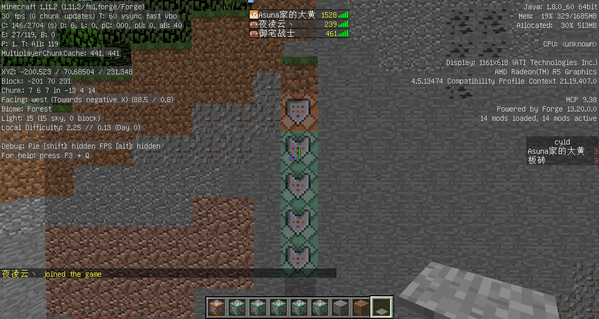# MC一种简单的可复制领地攻略

/scoreboard objectives add coin dummy （货币的计分板，关于经融系统，太多了，也就不用我介绍了）

/scoreboard objectives add cy dummy （领地号的计分板）

/scoreboard objectives add cyld dummy （是否在领地的计分板）/testfor @a[score_cy=1,score_cy_min=1,dx=21,dy=40,dz=21] （循环）（不受限制）（保持开启）

/scoreboard players set @a[score_cy=1,score_cy_min=1] cyld 1 （连锁）（条件限制）（保持开启）

/scoreboard players set @a[score_cy=1,score_cy_min=1] cyld 2 （脉冲）（不受限制）（红石控制）

/tp @a[dx=19,dy=38,dz=19,m=!0] x y z （循环）（不受限制）（红石控制）/gamemode 0 @a[score_cyld=1,score_cyld_min=1,m=!0] （循环）（不受限制）（保持开启）

/gamemode 2 @a[score_cyld=2,score_cyld_min=2,m=!2] （连锁）（不受限制）（保持开启）

/scoreboard players set @a[score_cyld=2,score_cyld_min=2] cyld 3 （连锁）（条件限制）（保持开启）/scoreboard players test @p[dx=0,dy=3,dz=0] coin 320 （脉冲）（不受限制）（红石控制）

/scoreboard players remove @p[dx=0,dy=4,dz=0] coin 320 （连锁）（条件限制）（保持开启）

/scoreboard players set @p[dx=0,dy=5,dz=0] cy 1 （连锁）（条件限制）（保持开启）

/tellraw @a {"text":"","extra":[{"text":"恭喜玩家","color":"green","bold":"true"},{"selector":"@p[dx=0,dy=6,dz=0,c=1]","color":"red","bold":"true"},{"text":"购买了 ","color":"green","bold":"true"},{"score":{"name":"@p[dx=0,dy=6,dz=0,c=1]","objective":"cy"},"bold":"true","color":"blue"},{"text":" 号领地！","color":"green","bold":"true"}]} （连锁）（条件限制）（保持开启）

/setblock ~ ~5 ~ minecraft:sea_lantern （连锁）（条件限制）（保持开启）